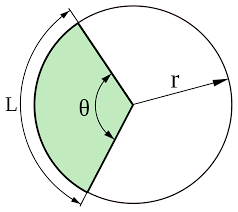## How to Calculate and Solve for the Area, Radius, Diameter and Angle of a Sector | The Calculator EncyclopediaThe image above is a sector.

To compute the area of a sector, two essential parameters is needed and this parameters are the radius of the sector (r) and the angle of the sector (θ). You can also use the diameter of the sector (d).

The formula for calculating the area of a sector:

Area of a sector = (θ/360)[πr²]

Where;

A = Area of the Sector
r = Radius of the Sector
θ = Angle of the Sector

Let’s solve an example:
Find the area of a sector when the radius of the sector is 7 cm and the angle of the sector is 9°

This implies that;

r = Radius of the sector = 7 cm
θ = Angle of the sector = 9°

A = θ / 360 x πr2
A = (9/360)[π x 7²]
A = 0.025 x π x 49
A = 3.848

Therefore, the area of the sector is 3.848 cm2.

Calculating the Area of a Sector using Diameter and Angle of the sector.

The formula is A = θ / 360 x πd2 / 4

Where;

θ = Angle of the sector
d = Diameter of the sector

Let’s solve an example;
Find the Area of a sector when the diameter of the sector is 12 cm and the angle of the sector is 18°.

This implies that;

d = Diameter of the sector = 12 cm
θ = Angle of the sector = 18°

A = θ / 360 x πd2 / 4
A = 18 / 360 x 3.142 (144) / 4
A = 18 / 360 x 452.448 / 4
A= 18 / 360 x 113.112
A= 0.05 x 113.112
A = 5.656

Therefore, the area of the sector with diameter is 5.656 cm

How to Calculate Angle of a Sector when Area of the Sector and Radius of the Sector is Given

θ = 360 (A) / πr2

where;

r = Radius of a sector
A = Area of a sector

Let’s solve an example;
Given that the area of a sector is 15 cm2 and the radius of the sector is 5 cm. Find the angle of the sector?

This implies that;
A = Area of the sector = 15 cm2
r = Radius of the sector = 5 cm

θ = 360 (A) / πr2
θ = 360 (15) / 3.142 (5)2
θ = 5400 / 3.142 (25)
θ = 5400 / 78.55
θ = 68.746

Therefore, the angle of the sector is 68.746°.

How to Calculate Angle of a Sector when Area of the Sector and Diameter of the Sector is Given

θ = 1440 (A) / πd2

where;

d = Diameter of a sector
A = Area of a sector

Let’s solve an example;
Given that the area of a sector is 22 cm2 and the diameter of the sector is 10 cm. Find the angle of the sector?

This implies that;
A = Area of the sector = 22 cm2
d = Diameter of the sector = 10 cm

θ = 1440 (A) / πr2
θ = 1440 (22) / 3.142 (10)2
θ = 31680 / 3.142 (100)
θ = 31680 / 314.2
θ = 100.88

Therefore, the angle of the sector is 100.88°.

How to Calculate Diameter of a Sector when Area of the Sector and Angle of the Sector is Given

d = √1440 (A) / πθ

where;

θ = Angle of a sector
A = Area of a sector

Let’s solve an example;
Given that the area of a sector is 24 cm2 and the angle of the sector is 10°. Find the diameter of the sector?

This implies that;
A = Area of the sector = 24 cm2
θ = Angle of the sector = 10°

d = √1440 (A) / πθ
d = √1440 (24) / 3.142 x 10
d = √34560 / 31.42
d = √1099.936
d = 33.165

Therefore, the diameter of the sector is 33.165 cm.

How to Calculate Radius of a Sector when Area of the Sector and Angle of the Sector is Given

r = √360 (A) / πθ

where;

θ = Angle of a sector
A = Area of a sector